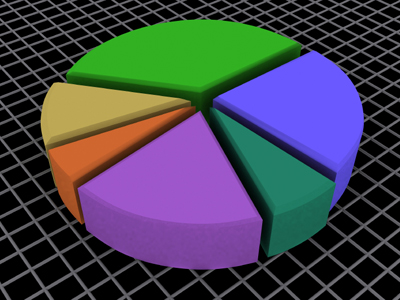A pie chart could be used in a spreadsheet to display data.

This Computer Science quiz is called 'Spreadsheets' and it has been written by teachers to help you if you are studying the subject at middle school. Playing educational quizzes is a fabulous way to learn if you are in the 6th, 7th or 8th grade - aged 11 to 14.

It costs only \$12.50 per month to play this quiz and over 3,500 others that help you with your school work. You can subscribe on the page at Join Us

Spreadsheets are interactive computer accounting programs that are very versatile. They are tables which you can tailor to any size you need and they can display any data you put into them in the form of numbers, text or even graphics. They are used by businesses and other organizations for many purposes, including accounting and record keeping. They are also used by people at home to work out their budgets, and by people at school to display the results of their science experiments as a graph. They are one of the most useful of all the different computer programs.

1.
A large piece of cloth spread over a flat surface
A software package used to organize information
A piece of A3 paper spread out so that you can see it clearly
A word processing file
Electronic spreadsheets can store, organize and display large quantities of data. They can also perform calculations
2.
What does it mean to 'format' a cell?
To make a cell bigger
To make a cell smaller
To insert a picture into a cell
To choose what type of information can be entered into a cell and how it is displayed
By right clicking on a cell and choosing 'format cells' from the menu, you can decide what type of information can be entered into that cell, whether currency (amounts of money), dates, text, numbers without decimals, numbers with two decimal places, etc. You can also format cells for font, color and borders
3.
A spreadsheet is divided into what?
Columns, rows and cells
Lines and squares
Parallel and diagonal lines
Helixes
Columns go vertically and rows horizontally. Where columns and rows meet is a box called a cell. These are the places information is entered
4.
Which symbol would you use in a formula for multiplication (to multiply two or more numbers)?
x
/
÷
*
An example of an instruction to multiply would look like this: =SUM(B6*B7)
5.
Which of these could a spreadsheet NOT be used for?
Creating a graph from your science investigation results
Calculating the cheapest cell phone package from a range of choices
Storing the test results for a class of year six pupils
You could use a word processor program to do your English writing homework
6.
On a spreadsheet, all cells are named. In the cell named B10, the 'B' refers to which of these?
The row where that cell is located
The type of information which can be entered into that cell
The column where that cell is located
The type of calculation which has been entered into that cell
The letters show in which column a cell is located, and the numbers show in which row the cell is located - this makes it very easy to find any named cell
7.
Which of these could be used in a spreadsheet to display your data?
A bar chart
A line graph
A pie chart
All of the above
To make a chart select the cells you want to use then click on the 'Chart' icon in the 'Insert' menu
8.
You are creating a spreadsheet to calculate the cost of a party. You want to know how much you will spend on pizzas. Why is it better to use a formula rather than working out the calculations yourself?
It will make it easier if you want to rearrange your spreadsheet
A formula will instantly calculate the change in cost if you need to change the price of the pizzas
The spreadsheet will be tidier if you use a formula
It is always faster to calculate in your head rather than to use a formula
A formula will instantly recalculate if you have to change any of the numbers which it uses. In this example, that could be the price of pizza, the number of people coming to the party, or even how many pieces of pizza each person will have
9.
Which of these is the correct formula for an addition?
=SUMB6+B7
B6+B7
=SUM(B6+B7)
=B6+B7
This formula would also work for an addition: =SUM(B6,B7)
10.
Which symbol would you use in a formula for division (dividing a number)?
x
/
÷
*
An example of an instruction to divide would look like this: =SUM(B6/B7)
Author:  Sheri Smith